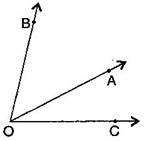### Basic Geometrical Ideas-Solutions Ex-4.3

CBSE Class –VI Mathematics
NCERT Solutions
Chapter 4
Basic Geometrical Ideas (Ex. 4.3)

Question 1. Name the angles in the given figure: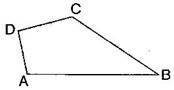Answer: There are four angles in given figure:
$\mathrm{\angle }$ABC, $\mathrm{\angle }$CDA, $\mathrm{\angle }$DAB, $\mathrm{\angle }$DCB
Question 2. In the given diagram, name the point(s):
(a) In the interior of $\mathrm{\angle }$DOE.
(b) In the exterior of $\mathrm{\angle }$EOF.
(c) On $\mathrm{\angle }$EOF.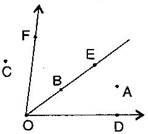Answer: (a) Point interior of $\mathrm{\angle }$DOE : A
(b) Points exterior of $\mathrm{\angle }$EOF : C, A, D
(c) Points on $\mathrm{\angle }$EOF : E, O, B, F
Question 3. Draw rough diagrams of two angles such that they have:
(a) One point in common.
(b) Two points in common.
(c) Three points in common.
(d) Four points in common.
(e) One ray in common.
(a)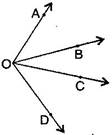(b)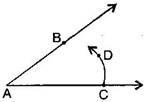(c)(d)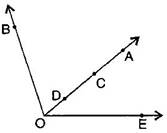(e)